# Torque Equation of DC Motor

Posted on

Torque equation of dc motor, simply and as Dr. Hugh d Young explains torque is the propensity of force to cause a rotational motion, and here in DC motor the torque develops between the armature and the stator, and it’s given by the product of the force and the radius at which the force acts.

The DC motor as we all know is a rotational machine, and torque of DC motor is a very important parameter in this concern, and it’s of utmost importance to understand the torque equation of DC motor for establishing its running characteristics.

# Torque Equation of DC Motor

When a DC machine is loaded either as a motor or as a generator, the rotor conductors carry current. These conductors lie in the magnetic field of the air gap. Thus each conductor experiences a force. The conductors lie near the surface of the rotor at a common radius from its center.

Hence torque is produced at the circumference of the rotor and rotor starts rotating. The term torque as best explained by Dr. Huge d Young is the quantitative measure of the tendency of a force to cause a rotational motion, or to bring about a change in rotational motion. It is in fact the moment of a force that produces or changes a rotational motion.

The equation of torque is given by,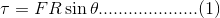Where,

• F is force in linear direction
• R is radius of the object being rotated, and
• θ is the angle, the force F is making with R vector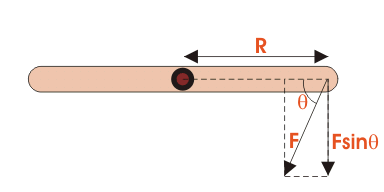## Basic Circuit Diagram of a DC motor

To establish the torque equation, let us first consider the basic circuit diagram of a DC motor, and its voltage equation.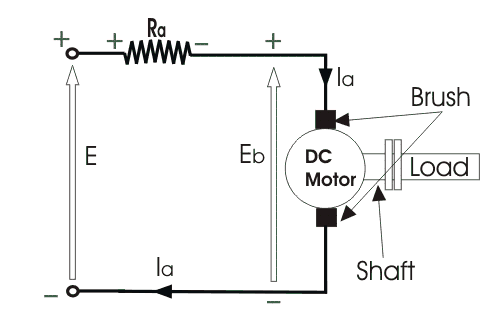Referring to the diagram beside, we can see, that if E is the supply voltage, Eb is the back emf produced and Ia, Ra are the armature current and armature resistance respectively then the voltage equation is given by,But keeping in mind that our purpose is to derive the torque equation of DC motor we multiply both sides of equation (2) by Ia.Now Ia2.Ra is the power loss due to heating of the armature coil, and the true effective mechanical power that is required to produce the desired torque of DC machine is given by,The mechanical power Pm is related to the electromagnetic torque Tg as,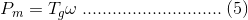Where, ω is speed in rad/sec. Now equating equation (4) and (5) we get,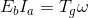Now for simplifying the torque equation of DC motor we substitute.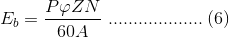Where,

• P is no of poles
• φ is flux per pole
• Z is no. of conductors
• A is no. of parallel paths, and
• N is the speed of the DC motor.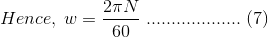Substituting equation (6) and (7) in equation (4), we get:## Electromagnetic Torque of DC Motor

The torque we so obtain, is known as the electromagnetic torque of DC motor, and subtracting the mechanical and rotational losses from it we get the mechanical torque. Therefore,This is the torque equation of DC motor. It can be further simplified as:Which is constant for a particular machine and therefore the torque of DC motor varies with only flux φ and armature current Ia. The Torque equation of a DC motor can also be explained considering the figure below.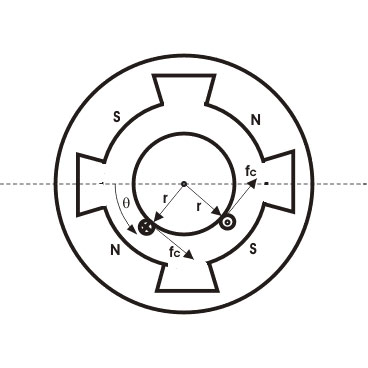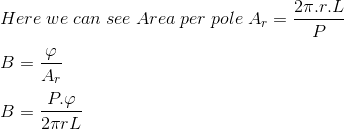Current/conductor Ic = Ia A

Therefore, force per conductor = fc = BLIa/A

Now torque Tc = fc. r = BLIa.r/A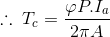Hence, the total torque developed of a DC machine is,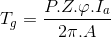This torque equation of DC motor can be further simplified as:Which is constant for a particular machine and therefore the torque of DC motor varies with only flux φ and armature current Ia.

### Torque Equation of DC Motor Conclusion

After going through the above portion of torque equation of dc motor we can now establish the torque equation. I hope you enjoy when reading this article, thank you.